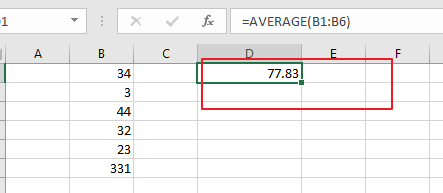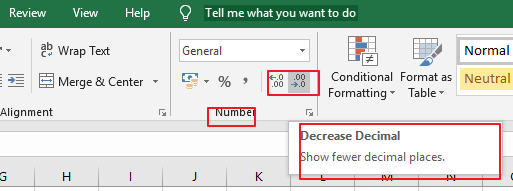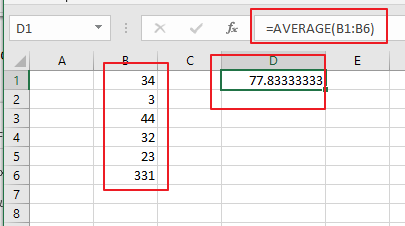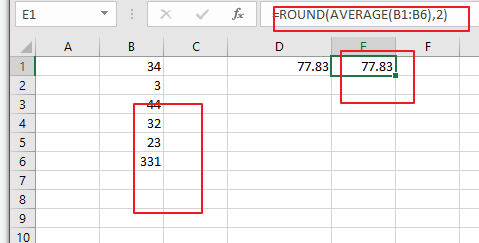# How to Round the Calculated Average result in Excel

This post will guide you how to use average and round functions at the same time to average a given range, and then round off the last result in Excel. How to use Round function to round the calculated average values with a formula in Excel.

Assuming that you have a list of data in range B1:B6 in which contain numeric values. And you can easily to calculate the average value with AVERAGE function. And the average value may contain two or more decimal places. And you want to round off this number to the specified number of decimal places. How to do it. You can use another function called ROUND. This post will show you tow methods to accomplish the result.

## Round the Calculated Average result with Decimal Command

To round the calculated average value for your data, you can use increase or decrease decimal command with the following steps:

Step1: select the range that to be averaged. Such as: B1:B6.

Step2: type the following formula based on the AVERAGE function to calculate the average value for the given range of cells:

`=AVERAGE(B1:B6)`

Type this formula into a blank cell and press Enter key on your keyboard. You would see that the average value has been calculated.Step3: select the calculated average value and go to Home tab, and click Increase decimal button or decrease decimal button under Number group.## Round the Calculated Average result with a Formula

You can also use a formula based on the ROUND function and AVERAGE function to accomplish the same result of rounding the calculated average result in Excel. Like the following formula:

`=ROUND(AVERAGE(B1:B6),2)`

Note: if you want to round off to the specified number of decimal places as you need, you just need to change the number 2 as you want.### Related Functions

• Excel Round function
The Excel INT function rounds a number to a specified number of digits. You can use the ROUND function to round to the left or right of the decimal point in Excel.The syntax of the ROUND function is as below:=ROUND (number, num_digits)…
• Excel AVERAGE function
The Excel AVERAGE function returns the average of the numbers that you provided.The syntax of the AVERAGE function is as below:=AVERAGE (number1,[number2],…)….
Related Posts

How to Average Last N Values in Multiple Columns

In Excel there are a lot of built-in functions, and AVERAGE is one of the most frequently used functions. In daily work, we may apply AVERAGE function together with some other functions to get average based on some conditions. In ...

How to Average Ignore Zero Number

AVERAGE function is a frequently used function in our office work. Except this basic function, Excel also provides some other Average related functions like AVERAGEIF, AVERAGEIFS. In this article, we will show you applying AVERAGEIF function to get average with ...

How to Average the Last N Values in Excel

In Excel there are a lot of built-in functions, and AVERAGE is one of the most frequently used functions. We can apply it to calculate the average of numbers from a given range reference. In daily work, we may apply ...

How to Calculate Average If Criteria Not Blank/Ignore Blank Cell

In daily work we often need to calculate the average of some numbers based on given conditions or criteria. To calculate average with criteria, we can apply AVERAGEIF of AVERAGEIFS function. AVERAGEIFS function can handle more than one group of ...

How to Calculate Average Ignore Non-Numeric Values and Errors

In daily work we often need to calculate the average of some numbers in a range for further analysis. Thus, we need to know some basic functions of calculate average in Excel. From now on, we will introduce you some ...

How to Average and Ignore Errors in Excel

This post will guide you how to average a list of values and ignoring any errors in the given range in Excel 2013/2016 or Excel office 365. You can use the AVERAGEIF function or AGGREGATE function to calculate the average ...

How to Calculate Average Ignore Blank and Zero Cells in Excel

Sometimes we need to calculate the average for a list or a range of numbers, but blank cells and zero cells may be also included in the selected range, so if these invalid cells are included, we often get error ...

How to Average Absolute Values in Excel

We can use AVERAGE function to calculate average of certain values. We can use ABS function to get absolute values for both positive number and negative number. If we want to get the average absolute values, we need to combine ...

How to Calculate Average among Multiple Different Worksheets in Excel

If we want to calculate the average for all numbers from multiple worksheets, how can we do calculate? Currently we may know the way to calculate the average for a selected range on one worksheet, this article will introduce you ...

How to Count the Average Between Two Dates in Excel

In our daily work, we usually have the problem of counting the average of a period. If the given dates are continuous, we can handle this situation very well, and if the dates are discontinuous, how do we count the ...

Sidebar